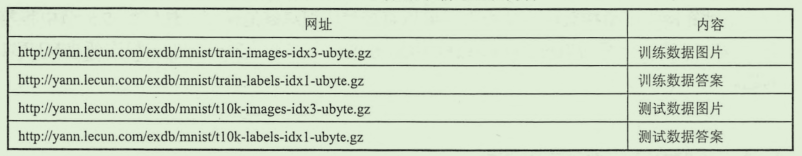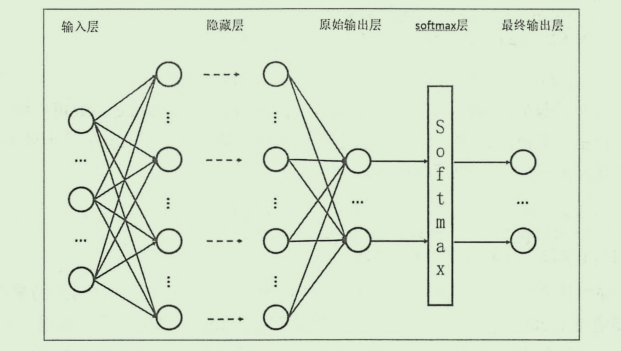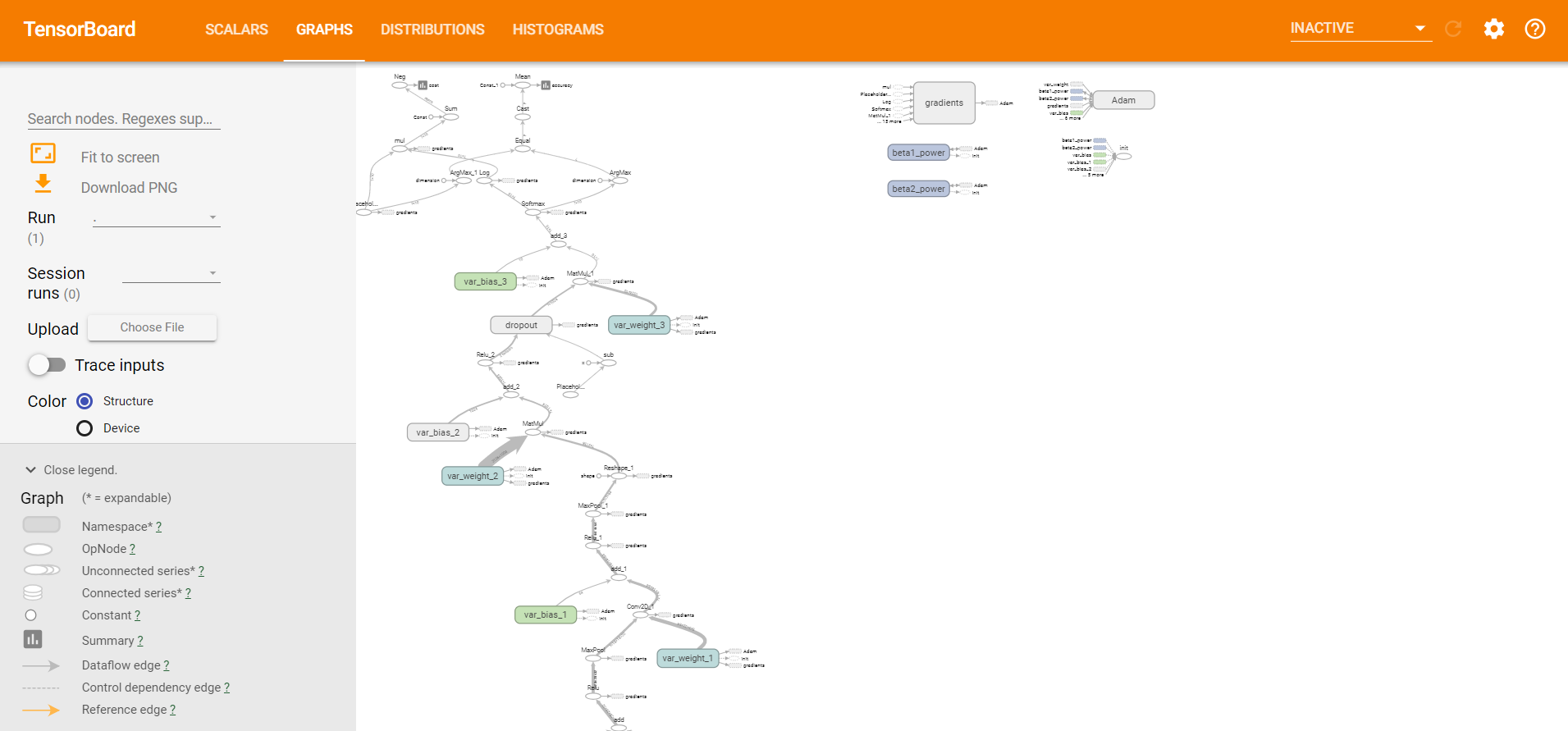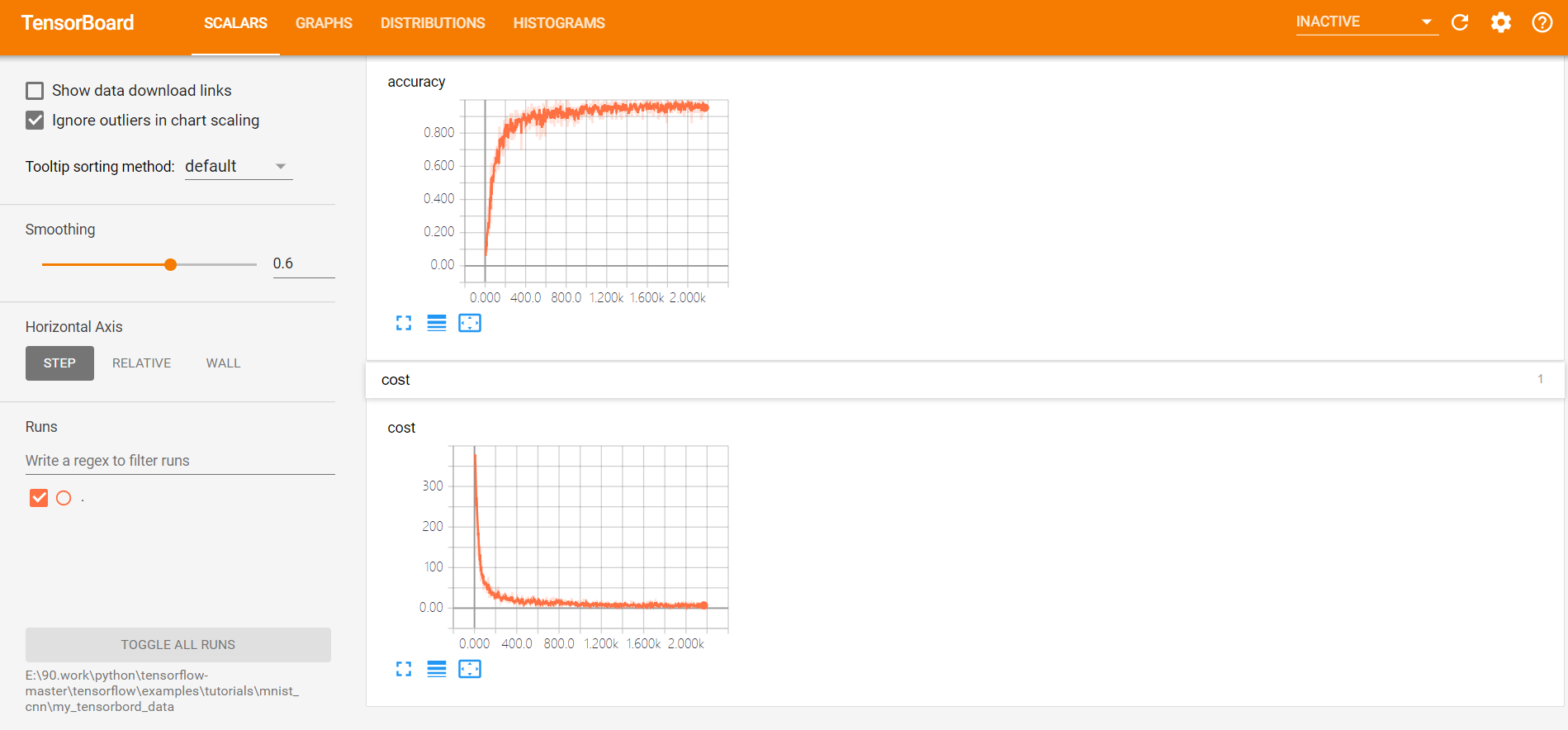• ## MNIST 手写数字识别（一）

万次阅读 多人点赞 2018-11-07 21:43:33
MNIST 手写数字识别模型建立与优化 本篇的主要内容有： TensorFlow 处理MNIST数据集的基本操作 建立一个基础的识别模型 介绍 SoftmaxSoftmaxSoftmax回归以及交叉熵等 MNIST是一个很有名的手写数字识别数据集（基本...
MNIST 手写数字识别模型建立与优化
本篇的主要内容有：

TensorFlow 处理MNIST数据集的基本操作
建立一个基础的识别模型
介绍 $Softmax$回归以及交叉熵等

MNIST是一个很有名的手写数字识别数据集（基本可以算是“Hello World”级别的了吧），我们要了解的情况是，对于每张图片，存储的方式是一个 28 * 28 的矩阵，但是我们在导入数据进行使用的时候会自动展平成 1 * 784（28 * 28）的向量，这在TensorFlow导入很方便，在使用命令下载数据之后，可以看到有四个数据集：模型
来看一个最基础的模型建立，首先了解TensoFlow对MNIST数据集的一些操作
1.TensorFlow 对MNIST数据集的操作
下载、导入
from tensorflow.examples.tutorials.mnist import input_data
# 第一次运行会自动下载到代码所在的路径下

# location 是保存的文件夹的名称

打印MNIST数据集的一些信息，通过这些我们就可以知道这些数据大致如何使用了
# 打印 mnist 的一些信息

from tensorflow.examples.tutorials.mnist import input_data

print("type of 'mnist is %s'" % (type(mnist)))
print("number of train data is %d" % mnist.train.num_examples)
print("number of test data is %d" % mnist.test.num_examples)

# 将所有的数据加载为这样的四个数组 方便之后的使用
trainimg = mnist.train.images
trainlabel = mnist.train.labels
testimg = mnist.test.images
testlabel = mnist.test.labels

print("Type of training is %s" % (type(trainimg)))
print("Type of trainlabel is %s" % (type(trainlabel)))
print("Type of testing is %s" % (type(testimg)))
print("Type of testing is %s" % (type(testlabel)))

输出结果：
type of 'mnist is <class 'tensorflow.contrib.learn.python.learn.datasets.base.Datasets'>'
number of train data is 55000    # 训练集共有55000条数据
number of test data is 10000     # 训练集有10000条数据
Type of training is <class 'numpy.ndarray'>    # 四个都是Numpy数组的类型
Type of trainlabel is <class 'numpy.ndarray'>
Type of testing is <class 'numpy.ndarray'>
Type of testing is <class 'numpy.ndarray'>

如果我们想看一看每条数据保存的图片是什么样子，可以使用 matplot()函数
# 接上面的代码

nsmaple = 5
randidx = np.random.randint(trainimg.shape, size=nsmaple)

for i in randidx:
curr_img = np.reshape(trainimg[i,:], (28, 28))  # 数据中保存的是 1*784 先reshape 成 28*28
curr_label = np.argmax(trainlabel[i, :])
plt.matshow(curr_img, cmap=plt.get_cmap('gray'))
plt.show()

通过上面的代码可以看出数据集中的一些特点，下面建立一个简单的模型来识别这些数字。
2.简单逻辑回归模型建立
显然，这是一个逻辑回归（分类）的问题，首先来建立一个最简单的模型，之后会逐渐地优化。分类模型一般会采用交叉熵方式作为损失函数，所以，对于这个模型的输出，首先使用 $Softmax$ 回归方式处理为概率分布，然后采用交叉熵作为损失函数，使用梯度下降的方式进行优化。
需要注意的地方直接卸载代码注释中了，只要根据这个过程走一遍，其实就很好理解了。（其实代码并不长，只是注释写的多，都记下来，防止以后忘了没处看 =_=||| ）。
import tensorflow as tf
from tensorflow.examples.tutorials.mnist import input_data

# 读入数据  ‘MNIST_data’ 是我保存数据的文件夹的名称

# 各种图片数据以及标签 images是图像数据  labels 是正确的结果
trainimg = mnist.train.images
trainlabels = mnist.train.labels
testimg = mnist.test.images
testlabels = mnist.test.labels

# 输入的数据 每张图片的大小是 28 * 28，在提供的数据集中已经被展平乘了 1 * 784（28 * 28）的向量
# 方便矩阵乘法处理
x = tf.placeholder(tf.float32, [None, 784])
# 输出的结果是对于每一张图输出的是 1*10 的向量，例如 [1, 0, 0, 0...]
# 只有一个数字是1 所在的索引表示预测数据
y = tf.placeholder(tf.float32, [None, 10])

# 模型参数
# 对于这样的全连接方式 某一层的参数矩阵的行数是输入数据的数量 ，列数是这一层的神经元个数
# 这一点用线性代数的思想考虑会比较好理解
W = tf.Variable(tf.zeros([784, 10]))
# 偏置
b = tf.Variable(tf.zeros())

# 建立模型 并使用softmax（）函数对输出的数据进行处理
# softmax（） 函数比较重要 后面写
# 这里注意理解一下 模型输出的actv的shape 后边会有用（n * 10, n时输入的数据的数量）
actv = tf.nn.softmax(tf.matmul(x, W) + b)

# 损失函数 使用交叉熵的方式  softmax（）函数与交叉熵一般都会结合使用
# clip_by_value()函数可以将数组整理在一个范围内，后面会具体解释
cost = tf.reduce_mean(-tf.reduce_sum(y * tf.log(tf.clip_by_value(actv, 1e-10, 1.0)), reduction_indices=1))

# 使用梯度下降的方法进行参数优化
learning_rate = 0.01

# 判断是否预测结果与正确结果是否一致
# 注意这里使用的函数的 argmax（）也就是比较的是索引 索引才体现了预测的是哪个数字
# 并且 softmax（）函数的输出不是[1, 0, 0...] 类似的数组 不会与正确的label相同
# pred 数组的输出是  [True, False, True...] 类似的
pred = tf.equal(tf.argmax(actv, 1), tf.argmax(y, 1))

# 计算正确率
# 上面看到pred数组的形式 使用cast转化为浮点数 则 True会被转化为 1.0, False 0.0
# 所以对这些数据求均值 就是正确率了（这个均值表示所有数据中有多少个1 -> True的数量 ->正确个数）
accr = tf.reduce_mean(tf.cast(pred, tf.float32))

init_op = tf.global_variables_initializer()

# 接下来要使用的一些常量 可能会自己根据情况调整所以都定义在这里
training_epochs = 50  # 一共要训练的轮数
batch_size = 100  # 每一批训练数据的数量
display_step = 5  # 用来比较、输出结果

with tf.Session() as sess:
sess.run(init_op)
# 对于每一轮训练
for epoch in range(training_epochs):
avg_cost = 0.
# 计算训练数据可以划分多少个batch大小的组
num_batch = int(mnist.train.num_examples / batch_size)

# 每一组每一组地训练
for i in range(num_batch):
# 这里地 mnist.train.next_batch()作用是：
# 第一次取1-10数据 第二次取 11-20 ... 类似这样
batch_xs, batch_ys = mnist.train.next_batch(batch_size)
# 运行模型进行训练
sess.run(optm, feed_dict={x: batch_xs, y: batch_ys})
# 如果觉得上面 feed_dict 的不方便 也可以提前写在外边
feeds = {x: batch_xs, y: batch_ys}
# 累计计算总的损失值
avg_cost += sess.run(cost, feed_dict=feeds) / num_batch

# 输出一些数据
if epoch % display_step == 0:
# 为了输出在训练集上的正确率本来应该使用全部的train数据 这里为了快一点就只用了部分数据
feed_train = {x: trainimg[1: 100], y: trainlabels[1: 100]}
# 在测试集上运行模型
feedt_test = {x: mnist.test.images, y: mnist.test.labels}
train_acc = sess.run(accr, feed_dict=feed_train)
test_acc = sess.run(accr, feed_dict=feedt_test)

print("Eppoch: %03d/%03d cost: %.9f train_acc: %.3f test_acc: %.3f" %
(epoch, training_epochs, avg_cost, train_acc, test_acc))
print("Done.")


输出结果：
Eppoch: 000/050 cost: 1.176410784 train_acc: 0.879 test_acc: 0.855
Eppoch: 005/050 cost: 0.440938284 train_acc: 0.919 test_acc: 0.896
Eppoch: 010/050 cost: 0.383333167 train_acc: 0.929 test_acc: 0.905
Eppoch: 015/050 cost: 0.357264753 train_acc: 0.939 test_acc: 0.909
Eppoch: 020/050 cost: 0.341510192 train_acc: 0.939 test_acc: 0.912
Eppoch: 025/050 cost: 0.330560439 train_acc: 0.939 test_acc: 0.914
Eppoch: 030/050 cost: 0.322391762 train_acc: 0.939 test_acc: 0.917
Eppoch: 035/050 cost: 0.315973353 train_acc: 0.939 test_acc: 0.917
Eppoch: 040/050 cost: 0.310739485 train_acc: 0.939 test_acc: 0.918
Eppoch: 045/050 cost: 0.306366821 train_acc: 0.939 test_acc: 0.919
Done.

可以看到，这个模型的正确率最后稳定在 92% 左右，不算高，毕竟只有一层处理。
下面来看几个重点：
$Softmax$ 回归
这个函数的作用是将一组数据转化为概率的形式,
函数表达式：
$Softmax(x_{j}) = \frac{exp(x_{j})}{\sum _{j} exp(x_{j})}$
$Softmax$回归可以将一组数据整理为一个概率分布，其实计算很简单，也很好理解，这里是用来处理模型的原本输出结果：这是因为模型原本的输出可能是$(1, 2, 3...)$这样形式，无法使用交叉熵的方式进行衡量，所以先进行一次处理，举个例子就是，对于一个向量 $(1, 2, ,3)$ 经过 $Softmax$ 回归之后就是 $(\frac{e^{1}}{e^{1}+e^{2}+e^{3}},\frac{e^{2}}{e^{1}+e^{2}+e^{3}},\frac{e^{3}}{e^{1}+e^{2}+e^{3}})$，这样就成为一个概率分布，方便接下来计算交叉熵了。
交叉熵的介绍
交叉熵（cross entropy）的概念取自信息论，刻画的是两个概率分布之间的距离，一般都会用在分类问题中，对于两个给定的概率分布 p 和 q，（注意：这里指的是 概率分布，不是单个的概率值，所以才会有下面公式中的求和运算）通过 q 来表示 p 的交叉熵表达为：
$H(p,q) = -\sum p(x)log \enspace q(x)$
这里还是要解释一下，使用交叉熵的前提：概率分布 p(X=x)必须要满足：
$\forall x p(X=x)\in [0,1] \enspace and \enspace \sum p(X=x)=1$
现在可以理解为什么要先使用$softmax$回归对输出地数据先进行处理了吧，本来模型对于一张图片的输出是不符合概率分布的，所以经过$softmax$回归转化之后，就可以使用交叉熵来衡量了。
如果通俗地理解交叉熵，可以理解为用给定的一个概率分布表达另一个概率分布的困难程度，如果两个概率分布越接近，那么显然这种困难程度就越小，那么交叉熵就会越小，回到MNIST中，我们知道对于某一张图片的label，也就是正确分类是这样的形式：(1, 0, 0, …) ，对于这张图片，我们的模型的输出可能是 (0.5, 0.3, 0.2) 这样的形式，那么计算交叉熵就是 $-(1 \times log(0.5) + 0 \times log(0.3) + 0 \times log(0.2))$ ，这样就计算出了交叉熵，在上面程序中 lost函数中就是这样计算的。这里还用到了一个函数 ： tf.clip_by_value()，这个函数是将数组中的值限定在一个范围内，上面程序的片段：
# 损失函数 使用交叉熵的方式  softmax（）函数与交叉熵一般都会结合使用
cost = tf.reduce_mean(-tf.reduce_sum(y * tf.log(tf.clip_by_value(actv, 1e-10, 1.0)), reduction_indices=1))

虽然模型的输出一般不会出现某个元素为0这种情况，但是这样并不保险，一旦出现actv中某个元素为0，根据交叉熵的计算，就会出现 log(0) 的情况，所以最好对这个数组加以限制，对于clip_by_value()函数，定义如下：
def clip_by_value(t: Any,           # 这个参数就是需要整理的数组
clip_value_min: Any,    # 最小值
clip_value_max: Any,    # 最大值
name: Any = None) ->
# 经过这个函数，数组中小于clip_value_min 的元素就会被替换为clip_value_min， 同样，超过的也会被替换
# 所以用在交叉熵中就保证了计算的合法

这样，很明显，交叉熵越小，也就说明模型地输出越接近正确的结果，这也是使用交叉熵描述损失函数地原因，接下来使用梯度下降（这里是）不断更新参数，找到最小地lost，就是最优的模型了。
以上~


展开全文• 官方Demo：... 1、源码 基于官方Demo增加注释和个人理解，增加tensorboard数据存储。 ...# Import the deep learning library import tensorflow as tf import ti...
官方Demo：https://www.w3cschool.cn/tensorflow_python/tensorflow_python-vj8528sp.html

1、源码

基于官方Demo增加注释和个人理解，增加tensorboard数据存储。


# Import the deep learning library
import tensorflow as tf
import time

#import input_data

from tensorflow.examples.tutorials.mnist import input_data

# placeholder：插入占位符
# 函数定义：placeholder(dtype, shape=None, name=None)
x = tf.placeholder("float", shape=[None, 784])
y_ = tf.placeholder("float", shape=[None, 10])

# tf.truncated_normal(shape, mean, stddev) :shape表示生成张量的维度，mean是均值，stddev是标准差。
# 这个函数产生正太分布，均值和标准差自己设定。
def weight_variable(shape):
with tf.variable_scope("var_weight"):
initial = tf.truncated_normal(shape, stddev=0.1)
var_weight = tf.Variable(initial)
tf.summary.histogram('var_weight',var_weight)
return var_weight

# tf.constant 创建一个常数张量,传入list或者数值来填充
def bias_variable(shape):
with tf.variable_scope("var_bias"):
initial = tf.constant(0.1, shape=shape)
var_bias = tf.Variable(initial)
tf.summary.histogram('var_bias',var_bias)
return var_bias

'''
函数名：tf.nn.conv2d(input, filter, strides, padding, use_cudnn_on_gpu=None, name=None)
函数说明：除去name参数用以指定该操作的name，与方法有关的一共五个参数：

第一个参数input：指需要做卷积的输入图像，它要求是一个Tensor，
具有[batch, in_height, in_width, in_channels]这样的shape，
具体含义是[训练时一个batch的图片数量, 图片高度, 图片宽度, 图像通道数]，注意这是一个4维的Tensor，
要求类型为float32和float64其中之一

第二个参数filter：相当于CNN中的卷积核，它要求是一个Tensor，
具有[filter_height, filter_width, in_channels, out_channels]这样的shape，
具体含义是[卷积核的高度，卷积核的宽度，图像通道数，卷积核个数]，要求类型与参数input相同，有一个地方需要注意，第三维in_channels，就是参数input的第四维

第三个参数strides：卷积时在图像每一维的步长，这是一个一维的向量，长度4

第五个参数：use_cudnn_on_gpu:bool类型，是否使用cudnn加速，默认为true

结果返回一个Tensor，这个输出，就是我们常说的feature map，shape仍然是[batch, height, width, channels]这种形式。

函数说明：将偏差项bias加到value上面

'''
def conv2d(x, W):
return tf.nn.conv2d(x, W, strides=[1, 1, 1, 1], padding='SAME')

'''
函数说明：
第一个参数value：需要池化的输入，一般池化层接在卷积层后面，
所以输入通常是feature map，依然是[batch, height, width, channels]这样的shape

第二个参数ksize：池化窗口的大小，取一个四维向量，
一般是[1, height, width, 1]，因为我们不想在batch和channels上做池化，所以这两个维度设为了1

第三个参数strides：和卷积类似，窗口在每一个维度上滑动的步长，一般也是[1, stride,stride, 1]

返回一个Tensor，类型不变，shape仍然是[batch, height, width, channels]这种形式
'''
def max_pool_2x2(x):
return tf.nn.max_pool(x, ksize=[1, 2, 2, 1],

W_conv1 = weight_variable([5, 5, 1, 32])
b_conv1 = bias_variable()

x_image = tf.reshape(x, [-1,28,28,1])

'''
函数名：tf.nn.relu(features, name = None)
函数说明：这个函数的作用是计算激活函数 relu，即 max(features, 0)。即将矩阵中每行的非最大值置0。
'''
h_conv1 = tf.nn.relu(conv2d(x_image, W_conv1) + b_conv1)
h_pool1 = max_pool_2x2(h_conv1)

W_conv2 = weight_variable([5, 5, 32, 64])
b_conv2 = bias_variable()

h_conv2 = tf.nn.relu(conv2d(h_pool1, W_conv2) + b_conv2)
h_pool2 = max_pool_2x2(h_conv2)

W_fc1 = weight_variable([7 * 7 * 64, 1024])
b_fc1 = bias_variable()

h_pool2_flat = tf.reshape(h_pool2, [-1, 7*7*64])
'''
函数说明：将矩阵 a 乘以矩阵 b,生成a * b

函数说明：这个函数是使x,和y两个参数的元素相加，返回的tensor数据类型和x的数据类型相同
'''
h_fc1 = tf.nn.relu(tf.matmul(h_pool2_flat, W_fc1) + b_fc1)

# tf.nn.dropout()是tensorflow里面为了防止或减轻过拟合而使用的函数，它一般用在全连接层
keep_prob = tf.placeholder("float")
h_fc1_drop = tf.nn.dropout(h_fc1, keep_prob)

W_fc2 = weight_variable([1024, 10])
b_fc2 = bias_variable()

'''
函数名：tf.nn.softmax(logits,axis=None,name=None,dim=None)
函数说明：计算softmax激活。
'''
y_conv=tf.nn.softmax(tf.matmul(h_fc1_drop, W_fc2) + b_fc2)

'''
函数名：
tf.nn.softmax_cross_entropy_with_logits(_sentinel=None,labels=None,
logits=None,dim=-1,name=None)
函数说明：计算logits和labels之间的softmax交叉熵

函数名：
reduce_mean(input_tensor,axis=None,keep_dims=False,name=None,reduction_indices=None)
函数说明：计算张量的各个维度上的元素的平均值.
'''

# 损失函数是目标类别和预测类别之间的交叉熵.
# 注意,tf.reduce_sum把minibatch里的每张图片的交叉熵值都加起来了.我们计算的交叉熵是指整个minibatch的.
cross_entropy = -tf.reduce_sum(y_*tf.log(y_conv))

'''
函数名:
函数说明：
相比于基础SGD算法，1.不容易陷于局部优点。2.速度更快
'''

'''
函数名：tf.argmax(input,axis)
函数说明：tf.argmax(input,axis)根据axis取值的不同返回每行或者每列最大值的索引。
axis=0的时候，比较每一列的元素，将每一列最大元素所在的索引记录下来，最后输出每一列最大元素所在的索引数组。
axis=1的时候，将每一行最大元素所在的索引记录下来，最后返回每一行最大元素所在的索引数组。

函数名：tf.equal(A, B)
函数说明：tf.equal(A, B)是对比这两个矩阵或者向量的相等的元素，
如果是相等的那就返回True，反正返回False，返回的值的矩阵维度和A是一样的

函数名：tf.cast(x,dtype,name=None)
函数说明：将x的数据格式转化成dtype
'''
correct_prediction = tf.equal(tf.argmax(y_conv,1), tf.argmax(y_,1))
# accuracy准确度
accuracy = tf.reduce_mean(tf.cast(correct_prediction, "float"))

'''
函数名：tf.summary.scalar(tags, values, collections=None, name=None)
函数说明：用来显示标量信息

函数名：tf.summary.merge(inputs,collections=None,name=None)
函数说明:合并标量信息
'''
cost = tf.summary.scalar("cost", cross_entropy)
training_accuracy = tf.summary.scalar("accuracy", accuracy)
#train_summary_merge = tf.summary.merge([cost,training_accuracy])
train_summary_merge = tf.summary.merge_all()

'''
batch是一个二维向量,包含50个训练数据信息
batch 训练数据图片，sharp ：(50,784)
batch 训练数据答案，sharp : (50,10)
'''

'''
tensor.eval()的意义和sess.run()一样，t.eval()等效于sess.run(t).
但是二者也有些微区别:run可以同时运行多个tensor;t.eval() 运行必须包含在默认的sess之内
'''

with tf.Session() as sess:
'''
函数名：tf.global_variables_initializer()
函数说明：初始化模型参数
'''
sess.run(tf.global_variables_initializer())

'''
函数名：tf.summary.FileWriter(self,logdir,graph=None,max_queue=10,flush_secs=120,graph_def=None)
函数说明：将汇总结果写入事件
'''
train_writer = tf.summary.FileWriter("./my_tensorbord_data", sess.graph)

for i in range(20000):
batch = mnist.train.next_batch(50)
if i%100 == 0:
# 在feed_dict中加入额外的参数keep_prob来控制 dropout 比例
train_accuracy = accuracy.eval(feed_dict={
x:batch, y_: batch, keep_prob: 1.0})
print ("step %d, training accuracy %g " % (i,train_accuracy))
summary, result = sess.run([train_summary_merge,train_step],feed_dict={x: batch, y_: batch, keep_prob: 0.5})

print ("test accuracy %g"%accuracy.eval(feed_dict={
x: mnist.test.images, y_: mnist.test.labels, keep_prob: 1.0}))


2、计算图3、准确率和损失率

电脑太挫，训练了将近2小时，测试数据准确率达99%。展开全文• ## 详解 MNIST 数据集

万次阅读 多人点赞 2017-07-17 20:41:35
MNIST 数据集已经是一个被”嚼烂”了的数据集, 很多教程都会对它”下手”, 几乎成为一个 “典范”. 不过有些人可能对它还不是很了解, 下面来介绍一下.MNIST 数据集可在 http://yann.lecun.com/exdb/mnist/ 获取, 它...
MNIST 数据集已经是一个被”嚼烂”了的数据集, 很多教程都会对它”下手”, 几乎成为一个 “典范”. 不过有些人可能对它还不是很了解, 下面来介绍一下.

MNIST 数据集可在 http://yann.lecun.com/exdb/mnist/ 获取, 它包含了四个部分:

Training set images: train-images-idx3-ubyte.gz (9.9 MB, 解压后 47 MB, 包含 60,000 个样本)
Training set labels: train-labels-idx1-ubyte.gz (29 KB, 解压后 60 KB, 包含 60,000 个标签)
Test set images: t10k-images-idx3-ubyte.gz (1.6 MB, 解压后 7.8 MB, 包含 10,000 个样本)
Test set labels: t10k-labels-idx1-ubyte.gz (5KB, 解压后 10 KB, 包含 10,000 个标签)
MNIST 数据集来自美国国家标准与技术研究所, National Institute of Standards and Technology (NIST). 训练集 (training set) 由来自 250 个不同人手写的数字构成, 其中 50% 是高中学生, 50% 来自人口普查局 (the Census Bureau) 的工作人员. 测试集(test set) 也是同样比例的手写数字数据.

不妨新建一个文件夹 – mnist, 将数据集下载到 mnist 以后, 解压即可:图片是以字节的形式进行存储, 我们需要把它们读取到 NumPy array 中, 以便训练和测试算法.

import os
import struct
import numpy as np

"""Load MNIST data from path"""
labels_path = os.path.join(path,
'%s-labels-idx1-ubyte'
% kind)
images_path = os.path.join(path,
'%s-images-idx3-ubyte'
% kind)
with open(labels_path, 'rb') as lbpath:
magic, n = struct.unpack('>II',
labels = np.fromfile(lbpath,
dtype=np.uint8)

with open(images_path, 'rb') as imgpath:
magic, num, rows, cols = struct.unpack('>IIII',
images = np.fromfile(imgpath,
dtype=np.uint8).reshape(len(labels), 784)

return images, labels

load_mnist 函数返回两个数组, 第一个是一个 n x m 维的 NumPy array(images), 这里的 n 是样本数(行数), m 是特征数(列数). 训练数据集包含 60,000 个样本, 测试数据集包含 10,000 样本. 在 MNIST 数据集中的每张图片由 28 x 28 个像素点构成, 每个像素点用一个灰度值表示. 在这里, 我们将 28 x 28 的像素展开为一个一维的行向量, 这些行向量就是图片数组里的行(每行 784 个值, 或者说每行就是代表了一张图片). load_mnist 函数返回的第二个数组(labels) 包含了相应的目标变量, 也就是手写数字的类标签(整数 0-9).

第一次见的话, 可能会觉得我们读取图片的方式有点奇怪:

magic, n = struct.unpack('>II', lbpath.read(8))
labels = np.fromfile(lbpath, dtype=np.uint8)

为了理解这两行代码, 我们先来看一下 MNIST 网站上对数据集的介绍:

TRAINING SET LABEL FILE (train-labels-idx1-ubyte):

[offset] [type]          [value]          [description]
0000     32 bit integer  0x00000801(2049) magic number (MSB first)
0004     32 bit integer  60000            number of items
0008     unsigned byte   ??               label
0009     unsigned byte   ??               label
........
xxxx     unsigned byte   ??               label
The labels values are 0 to 9.

通过使用上面两行代码, 我们首先读入 magic number, 它是一个文件协议的描述, 也是在我们调用 fromfile 方法将字节读入 NumPy array 之前在文件缓冲中的 item 数(n). 作为参数值传入 struct.unpack 的 >II 有两个部分:

>: 这是指大端(用来定义字节是如何存储的); 如果你还不知道什么是大端和小端, Endianness 是一个非常好的解释. (关于大小端, 更多内容可见<<深入理解计算机系统 – 2.1 节信息存储>>)
I: 这是指一个无符号整数.
通过执行下面的代码, 我们将会从刚刚解压 MNIST 数据集后的 mnist 目录下加载 60,000 个训练样本和 10,000 个测试样本.

为了了解 MNIST 中的图片看起来到底是个啥, 让我们来对它们进行可视化处理. 从 feature  matrix 中将 784-像素值 的向量 reshape 为之前的 28*28 的形状, 然后通过 matplotlib 的 imshow 函数进行绘制:

import matplotlib.pyplot as plt

fig, ax = plt.subplots(
nrows=2,
ncols=5,
sharex=True,
sharey=True, )

ax = ax.flatten()
for i in range(10):
img = X_train[y_train == i].reshape(28, 28)
ax[i].imshow(img, cmap='Greys', interpolation='nearest')

ax.set_xticks([])
ax.set_yticks([])
plt.tight_layout()
plt.show()

我们现在应该可以看到一个 2*5 的图片, 里面分别是 0-9 单个数字的图片.此外, 我们还可以绘制某一数字的多个样本图片, 来看一下这些手写样本到底有多不同:

fig, ax = plt.subplots(
nrows=5,
ncols=5,
sharex=True,
sharey=True, )

ax = ax.flatten()
for i in range(25):
img = X_train[y_train == 7][i].reshape(28, 28)
ax[i].imshow(img, cmap='Greys', interpolation='nearest')

ax.set_xticks([])
ax.set_yticks([])
plt.tight_layout()
plt.show()

执行上面的代码后, 我们应该看到数字 7 的 25 个不同形态:另外, 我们也可以选择将 MNIST 图片数据和标签保存为 CSV 文件, 这样就可以在不支持特殊的字节格式的程序中打开数据集. 但是, 有一点要说明, CSV 的文件格式将会占用更多的磁盘空间, 如下所示:

train_img.csv: 109.5 MB
train_labels.csv: 120 KB
test_img.csv: 18.3 MB
test_labels: 20 KB
如果我们打算保存这些 CSV 文件, 在将 MNIST 数据集加载入 NumPy array 以后, 我们应该执行下列代码:

np.savetxt('train_img.csv', X_train,
fmt='%i', delimiter=',')
np.savetxt('train_labels.csv', y_train,
fmt='%i', delimiter=',')
np.savetxt('test_img.csv', X_test,
fmt='%i', delimiter=',')
np.savetxt('test_labels.csv', y_test,
fmt='%i', delimiter=',')

一旦将数据集保存为 CSV 文件, 我们也可以用 NumPy 的 genfromtxt 函数重新将它们加载入程序中:

X_train = np.genfromtxt('train_img.csv',
dtype=int, delimiter=',')
y_train = np.genfromtxt('train_labels.csv',
dtype=int, delimiter=',')
X_test = np.genfromtxt('test_img.csv',
dtype=int, delimiter=',')
y_test = np.genfromtxt('test_labels.csv',
dtype=int, delimiter=',')

不过, 从 CSV 文件中加载 MNIST 数据将会显著发给更长的时间, 因此如果可能的话, 还是建议你维持数据集原有的字节格式.

参考:
- Book , Python Machine Learning.
展开全文python 机器学习 深度学习
• ## MNIST介绍

千次阅读 2018-05-15 10:39:37
MNIST这个教程的目标读者是对机器学习和TensorFlow都不太了解的新手。如果你已经了解MNIST和softmax回归(softmax regression)的相关知识，你可以阅读这个快速上手教程。当我们开始学习编程的时候，第一件事往往是...
MNIST这个教程的目标读者是对机器学习和TensorFlow都不太了解的新手。如果你已经了解MNIST和softmax回归(softmax regression)的相关知识，你可以阅读这个快速上手教程。当我们开始学习编程的时候，第一件事往往是学习打印"Hello World"。就好比编程入门有Hello World，机器学习入门有MNIST。MNIST是一个入门级的计算机视觉数据集，它包含各种手写数字图片：它也包含每一张图片对应的标签，告诉我们这个是数字几。比如，上面这四张图片的标签分别是5，0，4，1。在此教程中，我们将训练一个机器学习模型用于预测图片里面的数字。我们的目的不是要设计一个世界一流的复杂模型 -- 尽管我们会在之后给你源代码去实现一流的预测模型 -- 而是要介绍下如何使用TensorFlow。所以，我们这里会从一个很简单的数学模型开始，它叫做Softmax Regression。对应这个教程的实现代码很短，而且真正有意思的内容只包含在三行代码里面。但是，去理解包含在这些代码里面的设计思想是非常重要的：TensorFlow工作流程和机器学习的基本概念。因此，这个教程会很详细地介绍这些代码的实现原理。MNIST数据集MNIST数据集的官网是这份代码，然后用下面的代码导入到你的项目里面，也可以直接复制粘贴到你的代码文件里面。import tensorflow.examples.tutorials.mnist.input_data as input_data
mnist = input_data.read_data_sets("MNIST_data/", one_hot=True)下载下来的数据集被分成两部分：60000行的训练数据集（mnist.train）和10000行的测试数据集（mnist.test）。这样的切分很重要，在机器学习模型设计时必须有一个单独的测试数据集不用于训练而是用来评估这个模型的性能，从而更加容易把设计的模型推广到其他数据集上（泛化）。正如前面提到的一样，每一个MNIST数据单元有两部分组成：一张包含手写数字的图片和一个对应的标签。我们把这些图片设为“xs”，把这些标签设为“ys”。训练数据集和测试数据集都包含xs和ys，比如训练数据集的图片是 mnist.train.images ，训练数据集的标签是 mnist.train.labels。每一张图片包含28X28个像素点。我们可以用一个数字数组来表示这张图片：我们把这个数组展开成一个向量，长度是 28x28 = 784。如何展开这个数组（数字间的顺序）不重要，只要保持各个图片采用相同的方式展开。从这个角度来看，MNIST数据集的图片就是在784维向量空间里面的点, 并且拥有比较部分，其中有关于softmax的可交互式的可视化解释。）对于softmax回归模型可以用下面的图解释，对于输入的xs加权求和，再分别加上一个偏置量，最后再输入到softmax函数中：如果把它写成一个等式，我们可以得到：我们也可以用向量表示这个计算过程：用矩阵乘法和向量相加。这有助于提高计算效率。（也是一种更有效的思考方式）更进一步，可以写成更加紧凑的方式：实现回归模型为了用python实现高效的数值计算，我们通常会使用函数库，比如NumPy，会把类似矩阵乘法这样的复杂运算使用其他外部语言实现。不幸的是，从外部计算切换回Python的每一个操作，仍然是一个很大的开销。如果你用GPU来进行外部计算，这样的开销会更大。用分布式的计算方式，也会花费更多的资源用来传输数据。TensorFlow也把复杂的计算放在python之外完成，但是为了避免前面说的那些开销，它做了进一步完善。Tensorflow不单独地运行单一的复杂计算，而是让我们可以先用图描述一系列可交互的计算操作，然后全部一起在Python之外运行。（这样类似的运行方式，可以在不少的机器学习库中看到。）使用TensorFlow之前，首先导入它：import tensorflow as tf我们通过操作符号变量来描述这些可交互的操作单元，可以用下面的方式创建一个：x = tf.placeholder(tf.float32, [None, 784])x不是一个特定的值，而是一个占位符placeholder，我们在TensorFlow运行计算时输入这个值。我们希望能够输入任意数量的MNIST图像，每一张图展平成784维的向量。我们用2维的浮点数张量来表示这些图，这个张量的形状是[None，784 ]。（这里的None表示此张量的第一个维度可以是任何长度的。）我们的模型也需要权重值和偏置量，当然我们可以把它们当做是另外的输入（使用占位符），但TensorFlow有一个更好的方法来表示它们：Variable 。 一个Variable代表一个可修改的张量，存在在TensorFlow的用于描述交互性操作的图中。它们可以用于计算输入值，也可以在计算中被修改。对于各种机器学习应用，一般都会有模型参数，可以用Variable表示。W = tf.Variable(tf.zeros([784,10]))
b = tf.Variable(tf.zeros())我们赋予tf.Variable不同的初值来创建不同的Variable：在这里，我们都用全为零的张量来初始化W和b。因为我们要学习W和b的值，它们的初值可以随意设置。注意，W的维度是[784，10]，因为我们想要用784维的图片向量乘以它以得到一个10维的证据值向量，每一位对应不同数字类。b的形状是，所以我们可以直接把它加到输出上面。现在，我们可以实现我们的模型啦。只需要一行代码！y = tf.nn.softmax(tf.matmul(x,W) + b)首先，我们用tf.matmul(​​X，W)表示x乘以W，对应之前等式里面的，这里x是一个2维张量拥有多个输入。然后再加上b，把和输入到tf.nn.softmax函数里面。至此，我们先用了几行简短的代码来设置变量，然后只用了一行代码来定义我们的模型。TensorFlow不仅仅可以使softmax回归模型计算变得特别简单，它也用这种非常灵活的方式来描述其他各种数值计算，从机器学习模型对物理学模拟仿真模型。一旦被定义好之后，我们的模型就可以在不同的设备上运行：计算机的CPU，GPU，甚至是手机！训练模型为了训练我们的模型，我们首先需要定义一个指标来评估这个模型是好的。其实，在机器学习，我们通常定义指标来表示一个模型是坏的，这个指标称为成本（cost）或损失（loss），然后尽量最小化这个指标。但是，这两种方式是相同的。一个非常常见的，非常漂亮的成本函数是“交叉熵”（cross-entropy）。交叉熵产生于信息论里面的信息压缩编码技术，但是它后来演变成为从博弈论到机器学习等其他领域里的重要技术手段。它的定义如下：y 是我们预测的概率分布, y' 是实际的分布（我们输入的one-hot vector)。比较粗糙的理解是，交叉熵是用来衡量我们的预测用于描述真相的低效性。更详细的关于交叉熵的解释超出本教程的范畴，但是你很有必要好好反向传播算法(backpropagation algorithm)来有效地确定你的变量是如何影响你想要最小化的那个成本值的。然后，TensorFlow会用你选择的优化算法来不断地修改变量以降低成本。train_step = tf.train.GradientDescentOptimizer(0.01).minimize(cross_entropy)在这里，我们要求TensorFlow用梯度下降算法（gradient descent algorithm）以0.01的学习速率最小化交叉熵。梯度下降算法（gradient descent algorithm）是一个简单的学习过程，TensorFlow只需将每个变量一点点地往使成本不断降低的方向移动。当然TensorFlow也提供了其他许多优化算法：只要简单地调整一行代码就可以使用其他的算法。TensorFlow在这里实际上所做的是，它会在后台给描述你的计算的那张图里面增加一系列新的计算操作单元用于实现反向传播算法和梯度下降算法。然后，它返回给你的只是一个单一的操作，当运行这个操作时，它用梯度下降算法训练你的模型，微调你的变量，不断减少成本。现在，我们已经设置好了我们的模型。在运行计算之前，我们需要添加一个操作来初始化我们创建的变量：init = tf.initialize_all_variables()现在我们可以在一个Session里面启动我们的模型，并且初始化变量：sess = tf.Session()
sess.run(init)然后开始训练模型，这里我们让模型循环训练1000次！for i in range(1000):
batch_xs, batch_ys = mnist.train.next_batch(100)
sess.run(train_step, feed_dict={x: batch_xs, y_: batch_ys})该循环的每个步骤中，我们都会随机抓取训练数据中的100个批处理数据点，然后我们用这些数据点作为参数替换之前的占位符来运行train_step。使用一小部分的随机数据来进行训练被称为随机训练（stochastic training）- 在这里更确切的说是随机梯度下降训练。在理想情况下，我们希望用我们所有的数据来进行每一步的训练，因为这能给我们更好的训练结果，但显然这需要很大的计算开销。所以，每一次训练我们可以使用不同的数据子集，这样做既可以减少计算开销，又可以最大化地学习到数据集的总体特性。评估我们的模型那么我们的模型性能如何呢？首先让我们找出那些预测正确的标签。tf.argmax 是一个非常有用的函数，它能给出某个tensor对象在某一维上的其数据最大值所在的索引值。由于标签向量是由0,1组成，因此最大值1所在的索引位置就是类别标签，比如tf.argmax(y,1)返回的是模型对于任一输入x预测到的标签值，而 tf.argmax(y_,1) 代表正确的标签，我们可以用 tf.equal 来检测我们的预测是否真实标签匹配(索引位置一样表示匹配)。correct_prediction = tf.equal(tf.argmax(y,1), tf.argmax(y_,1))这行代码会给我们一组布尔值。为了确定正确预测项的比例，我们可以把布尔值转换成浮点数，然后取平均值。例如，[True, False, True, True] 会变成 [1,0,1,1] ，取平均值后得到 0.75.accuracy = tf.reduce_mean(tf.cast(correct_prediction, "float"))最后，我们计算所学习到的模型在测试数据集上面的正确率。print sess.run(accuracy, feed_dict={x: mnist.test.images, y_: mnist.test.labels})这个最终结果值应该大约是91%。这个结果好吗？嗯，并不太好。事实上，这个结果是很差的。这是因为我们仅仅使用了一个非常简单的模型。不过，做一些小小的改进，我们就可以得到97％的正确率。最好的模型甚至可以获得超过99.7％的准确率！（想了解更多信息，可以看看这个关于各种模型的<a rel="nofollow" href="http://rodrigob.github.io/are_we_there_yet/build/classification_datasets_results.html" "="" style="padding: 0px; margin: 0px; background-color: transparent; color: rgb(45, 133, 202);">性能对比列表。)比结果更重要的是，我们从这个模型中学习到的设计思想。不过，如果你仍然对这里的结果有点失望，可以查看下一个教程，在那里你可以学习如何用TensorFlow构建更加复杂的模型以获得更好的性能！
展开全文• MNIST 是机器学习领域的 Hello World 任务。由于各种原因，官方的开源实现往往会出现报错。本资源解决了此问题，并整合了：MNIST原始数据、经过转换的MNIST图片、一个简单的MNIST实现脚本，可完美运行。
• 1 读取本地MNIST数据，训练，保存模型 # -*- coding: utf-8 -*- """ Created on Thu Apr 9 21:10:34 2020 @author: tianx """ import numpy as np import os import gzip import keras from keras.models import ...
•python catboost 机器学习
• from keras.datasets import mnist from keras.utils import np_utils from keras.models import Sequential from keras.layers.core import Dense # 数据集 (X_train, y_train), (X_test, y_test) = mnist....TensorFlow Keras
• 例程1获取中间某一层的权重和偏置，并打印 from keras.models import Sequential,Model from keras.layers import Dense import numpy as np model = Sequential() model.add(Dense(32,activation="relu",input_...
• 需要安装cv2 ... 安装模型图片导出模块 udo pip install pydot sudo pip install graphviz pip install pydot-ng sudo apt-get install graphviz 安装h5py的命令如下（模型
• ## mnist数据集介绍

万次阅读 2018-10-07 15:01:17
参考博客： https://blog.csdn.net/tostq/article/details/51786265 参考代码: ... MINST数据库 MINST数据库是由Yann提供的手写数字数据库文件，其官方下载地址http://yann.lecun.com/exdb/mnist...
• ## 【MNIST】

千次阅读 2018-08-11 09:41:02
1. Normal Neural Network： 首先我用的是两层（input layer 和 output layer）的feed-forward的神经网络结构来训练数据, y = wx + b, 在输出层用的是softmax求概率，算loss用的是交叉熵的办法，选用梯度下降法来...
• MNIST数据集是一个入门级的计算机视觉数据集，数据集中都是美国中学生手写的数字，其中训练集包括6万张图片，测试集包括1万张图片，并且数字已经进行过预处理和格式化。
• ## 模仿mnist数据集制作自己的数据集

万次阅读 多人点赞 2017-08-05 20:18:28
模仿mnist数据集制作自己的数据集 最近看深度学习，用TensorFlow跑教程里的模型，发现教程里大多都是用mnist和cifar这两个数据集来演示的。想测试自己的数据集看看初步效果，于是就想套用现有的模型，将自己的数据集...制作
• ## 使用MNIST数据集

万次阅读 多人点赞 2018-06-09 17:11:23
网上有很多使用MINIST数据集的教程，要么太麻烦，要么需要翻墙下载，很慢。 在这里分享一下我找到的最方便的方法 1 下载数据集并解压。 法1 从MINIST官网中下载 法2 从这个百度云链接下载 没有网络限制...
• 1.一开始采用官网上利用input_data来...2.后面采用 keras加载mnist数据集，又会报出如下错误 URL fetch fail:http://googelsourc…………………………………… 解决办法：先将mnist数据集下载到本地，放到自己想...数据集
• mnist数据集是由深度学习大神 LeCun等人制作完成的数据集，mnist数据集也常认为是深度学习的“ Hello World！”。 官网：http://yann.lecun.com/exdb/mnist/ mnist数据集由6万张训练数据和1万张测试数据组成。 ...深度学习 MNIST
• 可从此页面获得的MNIST手写数字数据库的训练为60,000个示例，而测试为10,000个示例。它是NIST可提供的更大集合的子集。这些数字已进行尺寸规格化，并在固定尺寸的图像中居中。 对于那些想在实际数据上尝试学习...mnist
• 数据集下载网址：http://yann.lecun.com/exdb/mnist/ 下载后无需解压，将其放在一个文件夹下即可： 数据说明： 数据集常被分为2~3个部分 训练集(train set)：用来学习的一组例子，用来适应分类器的参数[即权重] ...
• MNIST的解压后的数据集，内含10000张测试集png图片，60000张训练集png图片，txt格式的测试集标签和训练集标签。 MNIST是最经典的手写数字数据集，但从数据集官网（http://yann.lecun.com/exdb/mnist/）下载下来后，......